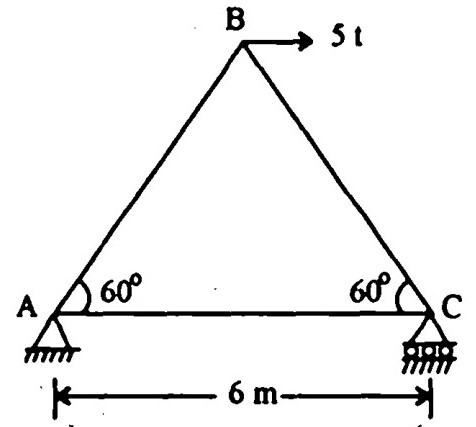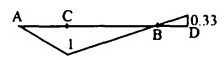Search

# Structural Analysis (101-120)

Updated: Apr 30, 2020

101.Which of the structures of Fig. will experience sway ?102.A symmetrical two-hinged parabolic arch when subjected to a uniformly distributed load on the entire horizontal span, is subject to

normal thrust alone

103.A two span continuous beam ABC is simply supported at A and C and is

continuous over support B. Span AB=6m, BC= 6m. The beam carries a udl of 2

t/m over both the spans. EI is constant for the entire beam. The fixed end moment at B in span BA or BC would be 9 t.m

104.A beam with a cantilevered arm BC supporting a freely supported end span

CD is shown in Fig. 12.29. Which one of Fig. represents the influence line

diagram for shear force at A ?105.The strain energy stored in a simply supported beam of span T and flexural,

rigidity EI due to a central concentrated load W is

W²l³/ 96 EI

106. In a two hinged arch an increase in temperature induces

maximum bending moment at the crown

107. The following methods are used for structural analysis:

1. Column analogy method

2. Kani's method

108. Figure shows a frame to be analyzed by moment distribution methodThe distribution factors for members, GF, GH and GD will be respectively

1/3, 1/3, 1/3

109.The frame shown in Fig. hassix unknown reaction components

110.The absolute maximum bending moment in a simply supported beam of span 20 m due to a moving udl of 4 t/m spanning over 5 m is

87.5 t-m at the midpoint

111.The influence line for horizontal thrust of a two-hinged parabolic arch of span 7' and rise 'h' will be as shown in112. Force in the member BC of the truss shown in Fig. is5 t, compressive

113.In respect of the beam and the given loading shown in Fig. 12.35, match list I

(span fixed-end moments) with list II (values) and select the correct answer

using the codes given below the lists114.The slope deflection equation of the member BC of the frame shown in Fig. is given by115.Which one of the following represents the correct influence line for bending

moment at point C for the beam shown in Fig. ?116.

1. The normal thrust at any section of the arch is the component of

interacting forces on the section along the tangent to the centre line of

the arch.

2. The radial shear at any section of the arch is the component of the

interacting forces on the section along the normal to the centre line of the

arch.

3. The intercept between a given arch and the linear arch at a section is

proportional to the bending moment at the section.

117. A symmetrical parabolic arch of span 20 metres and rise 5 metres is hinged at the springings. It supports a uniformly distributed load of 2 tonnes per metre run

of the span. The horizontal thrust in tonnes at each of the springings is 20

118. A beam AB is fixed at both ends and carries a uniformly distributed load of

intensity p per unit length run over its entire length. Due to some constructional defects, the end B is now reduced to a simple support. The percentage increase in bending moment at A is 50

119.120.A fixed beam AB is subjected to a triangular load varying from zero at end

A to w per unit length at end B. The ratio of fixed end moment at B to A will be

3/2